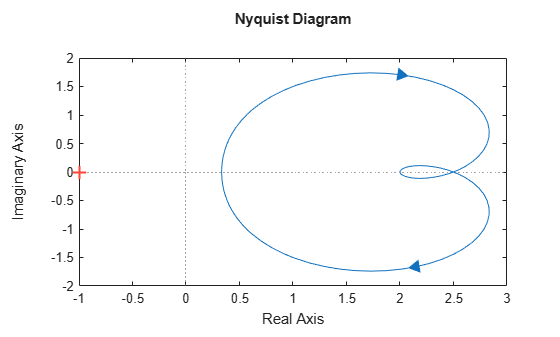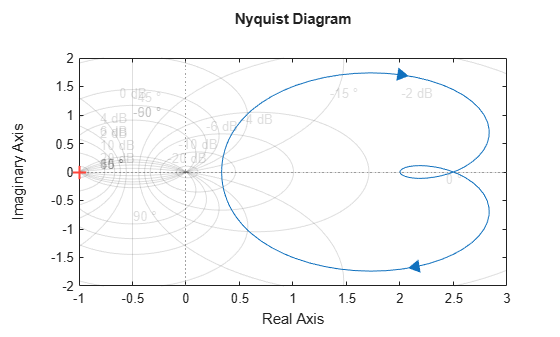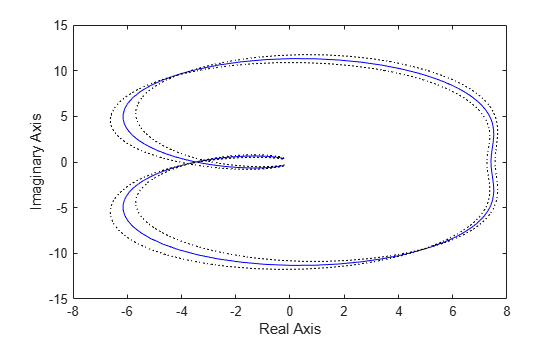# nyquist

Nyquist plot of frequency response

## Syntax

```nyquist(sys) nyquist(sys,w) nyquist(sys1,sys2,...,sysN) nyquist(sys1,sys2,...,sysN,w) nyquist(sys1,'PlotStyle1',...,sysN,'PlotStyleN') [re,im,w] = nyquist(sys) [re,im] = nyquist(sys,w) [re,im,w,sdre,sdim] = nyquist(sys) ```

## Description

`nyquist` creates a Nyquist plot of the frequency response of a dynamic system model. When invoked without left-hand arguments, `nyquist` produces a Nyquist plot on the screen. Nyquist plots are used to analyze system properties including gain margin, phase margin, and stability.

`nyquist(sys)` creates a Nyquist plot of a dynamic system `sys`. This model can be continuous or discrete, and SISO or MIMO. In the MIMO case, `nyquist` produces an array of Nyquist plots, each plot showing the response of one particular I/O channel. The frequency points are chosen automatically based on the system poles and zeros.

`nyquist(sys,w)` explicitly specifies the frequency range or frequency points to be used for the plot. To focus on a particular frequency interval, set ```w = {wmin,wmax}```. To use particular frequency points, set `w` to the vector of desired frequencies. Use `logspace` to generate logarithmically spaced frequency vectors. Frequencies must be in `rad/TimeUnit`, where `TimeUnit` is the time units of the input dynamic system, specified in the `TimeUnit` property of `sys`.

`nyquist(sys1,sys2,...,sysN)` or `nyquist(sys1,sys2,...,sysN,w)` superimposes the Nyquist plots of several LTI models on a single figure. All systems must have the same number of inputs and outputs, but may otherwise be a mix of continuous- and discrete-time systems. You can also specify a distinctive color, linestyle, and/or marker for each system plot with the syntax `nyquist(sys1,'PlotStyle1',...,sysN,'PlotStyleN')`.

`[re,im,w] = nyquist(sys)` and ```[re,im] = nyquist(sys,w)``` return the real and imaginary parts of the frequency response at the frequencies `w` (in `rad/TimeUnit`). `re` and `im` are 3-D arrays (see "Arguments" below for details).

`[re,im,w,sdre,sdim] = nyquist(sys)` also returns the standard deviations of `re` and `im` for the identified system `sys`.

## Arguments

The output arguments `re` and `im` are 3-D arrays with dimensions

For SISO systems, the scalars `re(1,1,k)` and `im(1,1,k)` are the real and imaginary parts of the response at the frequency ωk = w(k).

`$\begin{array}{l}\text{re}\left(1,1,k\right)=\mathrm{Re}\left(h\left(j{\omega }_{k}\right)\right)\\ \text{im}\left(1,1,k\right)=\mathrm{Im}\left(h\left(j{w}_{k}\right)\right)\end{array}$`

For MIMO systems with transfer function H(s), `re(:,:,k)` and `im(:,:,k)` give the real and imaginary parts of H(k) (both arrays with as many rows as outputs and as many columns as inputs). Thus,

`$\begin{array}{l}\text{re}\text{\hspace{0.17em}}\text{(i,}\text{\hspace{0.17em}}\text{j,}\text{\hspace{0.17em}}\text{k)}=\mathrm{Re}\left({h}_{ij}\left(j{\omega }_{k}\right)\right)\\ \text{im}\text{\hspace{0.17em}}\text{(i,}\text{\hspace{0.17em}}\text{j,}\text{\hspace{0.17em}}\text{k)}=\mathrm{Im}\left({h}_{ij}\left(j{\omega }_{k}\right)\right)\end{array}$`

where hij is the transfer function from input j to output i.

## Examples

collapse all

Create the following transfer function and plot its Nyquist response.

$\mathit{H}\left(\mathit{s}\right)=\frac{2{\mathit{s}}^{2}+5\mathit{s}+1}{{\mathit{s}}^{2}+2\mathit{s}+3}$.

```H = tf([2 5 1],[1 2 3]); nyquist(H)```The `nyquist` function can display a grid of M-circles, which are the contours of constant closed-loop magnitude. M-circles are defined as the locus of complex numbers where the following quantity is a constant value across frequency.

$\mathit{T}\left(\mathit{j}\omega \right)=|\frac{\mathit{G}\left(\mathit{j}\omega \right)}{1+\mathit{G}\left(\mathit{j}\omega \right)}|$.

Here, ω is the frequency in radians/`TimeUnit`, where `TimeUnit` is the system time units, and G is the collection of complex numbers that satisfy the constant magnitude requirement.

To display the grid of M-circles, right-click in the plot and select Grid. Alternatively, use the `grid` command.

`grid on`Compute the standard deviations of the real and imaginary parts of the frequency response of an identified model. Use this data to create a 3σ plot of the response uncertainty.

Load estimation data `z2`.

`load iddata2 z2;`

Identify a transfer function model using the data.

`sys_p = tfest(z2,2);`

Obtain the standard deviations for the real and imaginary parts of the frequency response for a set of 512 frequencies, `w`.

```w = linspace(-10*pi,10*pi,512); [re,im,wout,sdre,sdim] = nyquist(sys_p,w);```

Here `re` and `im` are the real and imaginary parts of the frequency response, and `sdre` and `sdim` are their standard deviations, respectively. The frequencies in `wout` are the same as the frequencies you specified in `w`.

Create a Nyquist plot showing the response and its 3σ uncertainty.

```re = squeeze(re); im = squeeze(im); sdre = squeeze(sdre); sdim = squeeze(sdim); plot(re,im,'b',re+3*sdre,im+3*sdim,'k:',re-3*sdre,im-3*sdim,'k:') xlabel('Real Axis'); ylabel('Imaginary Axis');```## Tips

• You can change the properties of your plot, for example the units. For information on the ways to change properties of your plots, see Ways to Customize Plots. For the most flexibility in customizing plot properties, use the `nyquistplot` command instead of `nyquist`.

• There are two zoom options available from the right-click menu that apply specifically to Nyquist plots:

• Full View — Clips unbounded branches of the Nyquist plot, but still includes the critical point (–1, 0).

• Zoom on (-1,0) — Zooms around the critical point (–1,0). (To access critical-point zoom programmatically, use `nyquistplot` instead.)

• To activate data markers that display the real and imaginary values at a given frequency, click anywhere on the curve. The following figure shows a `nyquist` plot with a data marker.## Algorithms

See `bode`.